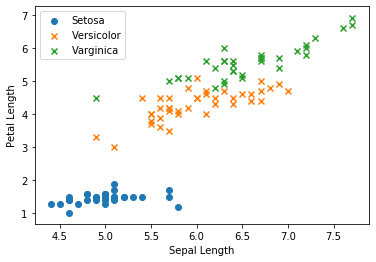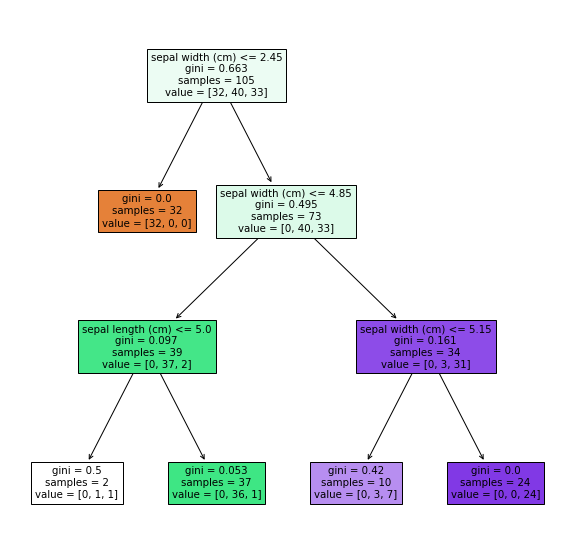# [PYTHON] Decision tree (load_iris)

## ■ Introduction

This time, we will summarize the implementation-plot of the decision tree.

[Target readers] ・ Those who want to learn the basic code in decision trees ・ I don't know the theory in detail, but those who want to see the implementation and give an image, etc.

## 1. Preparation of module

First, import the required modules.

``````
import numpy as np
import pandas as pd
import matplotlib.pyplot as plt

from sklearn.datasets import load_iris
from sklearn.model_selection import train_test_split
from sklearn.tree import DecisionTreeClassifier
from sklearn.tree import plot_tree
``````

## 2. Data preparation Use the load_iris dataset.
``````
X, y = iris.data[:, [0, 2]], iris.target

print(X.shape)
print(y.shape)

# (150, 2)
# (150,)
``````

Divide into train and test data.

``````
X_train, X_test, y_train, y_test = train_test_split(X, y, test_size = 0.3, random_state = 123)

print(X_train.shape)
print(y_train.shape)
print(X_test.shape)
print(y_train.shape)

# (105, 2)
# (105,)
# (45, 2)
# (45,)
``````

In the decision tree, individual features are processed independently, and data division is scale-independent. No normalization or standardization is required.

## 3. Data visualization

Before modeling, let's plot the data.

``````
fig, ax = plt.subplots()

ax.scatter(X_train[y_train == 0, 0], X_train[y_train == 0, 1],
marker = 'o', label = 'Setosa')

ax.scatter(X_train[y_train == 1, 0], X_train[y_train == 1, 1],
marker = 'x', label = 'Versicolor')

ax.scatter(X_train[y_train == 2, 0], X_train[y_train == 2, 1],
marker = 'x', label = 'Varginica')

ax.set_xlabel('Sepal Length')
ax.set_ylabel('Petal Length')
ax.legend(loc = 'best')

plt.show()
``````## 4. Creating a model

Create a model of the decision tree.

``````
tree = DecisionTreeClassifier(max_depth = 3)
tree.fit(X_train, y_train)

'''
DecisionTreeClassifier(ccp_alpha=0.0, class_weight=None, criterion='gini',
max_depth=3, max_features=None, max_leaf_nodes=None,
min_impurity_decrease=0.0, min_impurity_split=None,
min_samples_leaf=1, min_samples_split=2,
min_weight_fraction_leaf=0.0, presort='deprecated',
random_state=None, splitter='best')

'''
``````

At the same time, I will also visualize it.

``````
fig, ax = plt.subplots(figsize=(10, 10))
plot_tree(tree, feature_names=iris.feature_names, filled=True)
plt.show()
``````## 5. Output of predicted value

Make predictions for test data.

``````
y_pred = tree.predict(X_test)

print(y_pred[:10])
print(y_test[:10])

# [2 2 2 1 0 1 1 0 0 1]
# [1 2 2 1 0 2 1 0 0 1]
``````

0：Setosa　1：Versicolor　2：Verginica

## 6. Performance evaluation

Find the correct answer rate in this classification prediction.

``````
print('{:.3f}'.format(tree.score(X_test, y_test)))

# 0.956
``````

## ■ Finally This time, based on the steps 1 to 6 above, we created and evaluated a model of the decision tree. We hope that it will be of some help to beginners.
## ■ References ・ [New data analysis textbook using Python](https://www.shoeisha.co.jp/book/detail/9784798158341)